• vgg16迁移学习
多人点赞
2021-09-05 11:25:48

# 一、导入相关库

import numpy as np
import tensorflow as tf
from tensorflow.keras.preprocessing.image import ImageDataGenerator
from tensorflow.keras.utils import to_categorical
from tensorflow.keras.models import Sequential
from tensorflow.keras.layers import Dense,Dropout,Conv2D,MaxPool2D,Flatten
from tensorflow.keras.optimizers import Adam
import matplotlib.pyplot as plt
from tensorflow.keras.callbacks import LearningRateScheduler
from tensorflow.keras.callbacks import EarlyStopping
import json
from tensorflow.keras.applications.vgg16 import VGG16


# 定义超参数

# 类别数
num_classes = 2
# 批次大小
batch_size = 8
# 周期数
epochs = 10
# 图片大小
image_size = 224


# 数据增加

# 训练集数据进行数据增强
train_datagen = ImageDataGenerator(
rotation_range = 20,     # 随机旋转度数
width_shift_range = 0.1, # 随机水平平移
height_shift_range = 0.1,# 随机竖直平移
rescale = 1/255,         # 数据归一化
shear_range = 10,       # 随机错切变换
zoom_range = 0.1,        # 随机放大
horizontal_flip = True,  # 水平翻转
brightness_range=(0.7, 1.3), # 亮度变化
fill_mode = 'nearest',   # 填充方式
)
# 测试集数据只需要归一化就可以
test_datagen = ImageDataGenerator(
rescale = 1/255,         # 数据归一化
)


# 构造生成器

# 训练集数据生成器，可以在训练时自动产生数据进行训练
# 从'data/train'获得训练集数据
# 获得数据后会把图片resize为image_size×image_size的大小
# generator每次会产生batch_size个数据
train_generator = train_datagen.flow_from_directory(
'data/train',
target_size=(image_size,image_size),
batch_size=batch_size,
)

# 测试集数据生成器
test_generator = test_datagen.flow_from_directory(
'data/test',
target_size=(image_size,image_size),
batch_size=batch_size,
)


# 做标签


label = train_generator.class_indices
label = dict(zip(label.values(),label.keys()))
with open('label_cat_dog.json','w',encoding='utf-8') as f:
json.dump(label, f)

# 字典的键为17个文件夹的名字，值为对应的分类编号
train_generator.class_indices


# 查看模型

# 载入使用imagenet训练好的预训练模型
# include_top=True表示模型包含全连接层
# include_top=False表示模型不包含全连接层
vgg16 = VGG16(weights='imagenet',include_top=False, input_shape=(image_size,image_size,3))
vgg16.summary()


# 改变VGG16的全连接层

# 搭建全连接层，连接在VGG16模型后面
# 我们主要是利用VGG16卷积网络已经训练好的特征提取能力来提取特征
# 然后搭建新的全连接层来进行新图片类型的分类
top_model = Sequential()

model = Sequential()
model.summary()


# 构造回调函数

early_stoping = EarlyStopping(monitor='val_accuracy', patience=3, verbose=1)


## 调节学习率

# 学习率调节函数，逐渐减小学习率
if epoch<=10:
lr = 1e-5
else:
lr = 1e-6
return lr


# 训练模型

# 定义优化器

callbacks = []

# 定义优化器，loss function，训练过程中计算准确率

# Tensorflow2.1版本之前可以使用fit_generator训练模型
# history = model.fit_generator(train_generator,steps_per_epoch=len(train_generator),epochs=epochs,validation_data=test_generator,validation_steps=len(test_generator))

# Tensorflow2.1版本(包括2.1)之后可以直接使用fit训练模型
history = model.fit(x=train_generator,
epochs=epochs,
validation_data=test_generator,
callbacks=[early_stoping])


# 准确率曲线

# 画出训练集准确率曲线图
plt.plot(np.arange(epochs),history.history['accuracy'],c='b',label='train_accuracy')
# 画出验证集准确率曲线图
plt.plot(np.arange(epochs),history.history['val_accuracy'],c='y',label='val_accuracy')
# 图例
plt.legend()
# x坐标描述
plt.xlabel('epochs')
# y坐标描述
plt.ylabel('accuracy')
# 显示图像
plt.show()
# 模型保存
# model.save('vgg16.h5')
model.save('cat_dog_model', save_format='tf')python
更多相关内容
• vgg16迁移学习transfer_learing-附件资源
• # add the model on top of the convolutional base model = Model(inputs=base_model.input, outputs=model(base_model.output)) # VGG16模型与自己构建的模型合并 # 保持VGG16的前15层权值不变，即在训练过程中不...
#代码参考网上博客
import random

from imutils import paths
from keras.optimizers import SGD
from keras_preprocessing.image import load_img, img_to_array
from tensorflow.python.keras.utils import get_file
import gzip
import numpy as np
import keras
from keras.datasets import cifar10
from keras.preprocessing.image import ImageDataGenerator
from keras.models import Sequential, Model
from keras.layers import Dense, Dropout, Activation, Flatten
from keras.layers import Conv2D, MaxPooling2D
import os
from keras import applications, Input
import cv2
import tensorflow as tf

#用自己的数据集实现4分类
# image = cv2.resize(image, (224, 224))
# print(type(image))
return image

# 获得模型用数据结构
imagePaths = list(paths.list_images(datapath))
random.shuffle(imagePaths)
labels = [int(i.split('\\')[-1]) for i in imagePaths]
# print(labels)
images = np.array([load_images(i) for i in imagePaths])
return (images, labels)

# include_top = False表明我们迁移除顶层以外的其余网络结构到自己的模型中
img_width, img_height = 224, 224
train_data_dir = 'D:\PPG-picture\HR_dataset_5\\train\\'
test_data_dir = 'D:\PPG-picture\HR_dataset_5\\test\\'
(x_train, y_train) = load_data_split1(train_data_dir)
(x_test, y_test) = load_data_split1(test_data_dir)
# 将类别弄成独热编码
y_train = keras.utils.to_categorical(y_train, 4)
y_test = keras.utils.to_categorical(y_test, 4)

print('[INFO] initializing model......')
# 即每次训练在训练集中取batchsize个样本训练；
batch_size = 32
num_classes = 4
# 完整的数据集在同样的神经网络中传递5次
epochs = 50
data_augmentation = False# 图像增强
# num_predictions = 20
# 模型保存：
save_dir = os.path.join(os.getcwd(), 'saved_models_transfer_learning')
print('模型保存路径:', save_dir)
model_name = 'keras_fashion_transfer_learning_trained_model1.h5'

print('[INFO] initializing model......')
base_model = applications.VGG16(include_top=False, weights='imagenet',
input_tensor=Input(shape=(img_width, img_height, 3)))  # 第一层需要指出图像的大小
base_model.summary()
model = Sequential()
print('基本模型的输出：', base_model.output)
# add the model on top of the convolutional base
model = Model(inputs=base_model.input, outputs=model(base_model.output))  # VGG16模型与自己构建的模型合并
# 保持VGG16的前15层权值不变，即在训练过程中不训练
for layer in model.layers[:15]:
layer.trainable = False

# 初始化 RMSprop 优化器
opt = keras.optimizers.rmsprop(lr=0.0001, decay=1e-6)
sgd = SGD(lr=0.0001, momentum=0.9)
# Let's train the model using RMSprop
model.compile(loss='categorical_crossentropy',
optimizer=sgd,
# optimizer=sgd,
metrics=['accuracy'])

print('[INFO] training model')

if not data_augmentation:
print('Not using data augmentation.')
history = model.fit(x_train, y_train,
batch_size=batch_size,
epochs=epochs,
validation_data=(x_test, y_test),
shuffle=True)
else:
print('Using real-time data augmentation.')
# This will do preprocessing and realtime data augmentation:
datagen = ImageDataGenerator(
featurewise_center=False,  # set input mean to 0 over the dataset
samplewise_center=False,  # set each sample mean to 0
featurewise_std_normalization=False,  # divide inputs by std of the dataset
samplewise_std_normalization=False,  # divide each input by its std
zca_whitening=False,  # apply ZCA whitening
zca_epsilon=1e-06,  # epsilon for ZCA whitening
rotation_range=0,  # randomly rotate images in the range (degrees, 0 to 180)
# randomly shift images horizontally (fraction of total width)
width_shift_range=0.1,
# randomly shift images vertically (fraction of total height)
height_shift_range=0.1,
shear_range=0.,  # set range for random shear
zoom_range=0.,  # set range for random zoom
channel_shift_range=0.,  # set range for random channel shifts
# set mode for filling points outside the input boundaries
fill_mode='nearest',
cval=0.,  # value used for fill_mode = "constant"
horizontal_flip=False,  # randomly flip images
vertical_flip=False,  # randomly flip images
# set rescaling factor (applied before any other transformation)
rescale=None,
# set function that will be applied on each input
preprocessing_function=None,
# image data format, either "channels_first" or "channels_last"
data_format=None,
# fraction of images reserved for validation (strictly between 0 and 1)
)
#测试上面的ImageDataGenerator的效果
x=img_to_array(img1)
x = x.reshape((1,) + x.shape)
i = 0
for batch in datagen.flow(x, batch_size=1,
save_to_dir='D:\PPG-picture\HR_dataset_5\\', save_prefix='test', save_format='jpeg'):
i += 1
if i > 20:
break

# Compute quantities required for feature-wise normalization
# (std, mean, and principal components if ZCA whitening is applied).
datagen.fit(x_train)
print(x_train.shape // batch_size)  # 取整
print(x_train.shape / batch_size)  # 保留小数
# Fit the model on the batches generated by datagen.flow().
history = model.fit_generator(datagen.flow(x_train, y_train,  # 按batch_size大小从x,y生成增强数据
batch_size=batch_size),
# flow_from_directory()从路径生成增强数据,和flow方法相比最大的优点在于不用
# 一次将所有的数据读入内存当中,这样减小内存压力，这样不会发生OOM
epochs=epochs,
steps_per_epoch=x_train.shape // batch_size,
validation_data=(x_test, y_test),
workers=10  # 在使用基于进程的线程时，最多需要启动的进程数量。
)

model.summary()
# Save model and weights
if not os.path.isdir(save_dir):
os.makedirs(save_dir)
model_path = os.path.join(save_dir, model_name)
model.save(model_path)
print('Saved trained model at %s ' % model_path)

import matplotlib.pyplot as plt

# 绘制训练 & 验证的准确率值
plt.plot(history.history['acc'])
plt.plot(history.history['val_acc'])
plt.title('Model accuracy')
plt.ylabel('Accuracy')
plt.xlabel('Epoch')
plt.legend(['Train', 'Valid'], loc='upper left')
plt.show()

# 绘制训练 & 验证的损失值
plt.plot(history.history['loss'])
plt.plot(history.history['val_loss'])
plt.title('Model loss')
plt.ylabel('Loss')
plt.xlabel('Epoch')
plt.legend(['Train', 'Valid'], loc='upper left')
plt.show()


展开全文神经网络
• VGG16分类网络,可以用于分类问题，采用了迁移学习
• VGG16迁移学习实现 本文讨论迁移学习，它是一个非常强大的深度学习技术，在不同领域有很多应用。动机很简单，可以打个比方来解释。假设想学习一种新的语言，比如西班牙语，那么从已经掌握的另一种语言（比如英语）学...

VGG16迁移学习实现
本文讨论迁移学习，它是一个非常强大的深度学习技术，在不同领域有很多应用。动机很简单，可以打个比方来解释。假设想学习一种新的语言，比如西班牙语，那么从已经掌握的另一种语言（比如英语）学起，可能是有用的。

按照这种思路，计算机视觉研究人员通常使用预训练 CNN 来生成新任务的表示，其中数据集可能不够大，无法从头开始训练整个 CNN。另一个常见的策略是采用在 ImageNet 上预训练好的网络，然后通过微调整个网络来适应新任务。

这里提出的例子受启于 Francois Chollet 写的关于 Keras 的一个非常有名的博客（https://blog.keras.io/building-powerful-image-classification-models-using-very-little-data.html）。
这个想法是使用在像 ImageNet 这样的大型数据集上预先训练的 VGG16 网络。注意，训练的计算量可能相当大，因此使用已经预训练的网络是有意义的：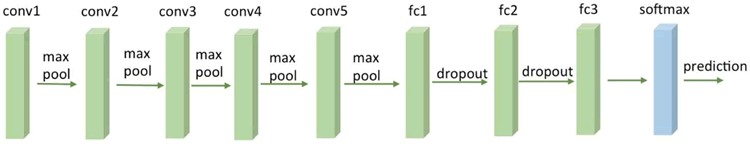图 1. 一个 VGG16 网络
如何使用 VGG16 呢？Keras 使其变得容易，因为有一个标准的 VGG16 模型可以作为一个库来使用，预先计算好的权重会自动下载。注意，这里省略了最后一层，并将其替换为自定义层，该层将在预定义的 VGG16 的顶部进行微调。

例如，下面将学习如何分类 Kaggle 提供的狗和猫的图片：

1. 从 Kaggle（https://www.kaggle.com/c/dogs-vs-cats/data）下载狗和猫的数据，并创建一个包含两个子目录（train 和 validation）的数据目录，每个子目录有两个额外的子目录，分别是 dogs 和 cats。

2. 导入稍后将用于计算的 Keras 模块，并保存一些有用的常量：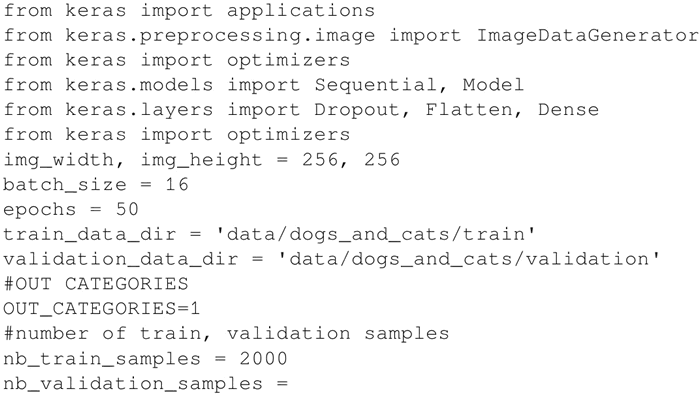3. 加载 ImageNet 上预训练的 VGG16 网络，省略最后一层，因为这里将在预建的 VGG16 网络的顶部添加自定义分类网络，并替换原来 VGG16 的分类层：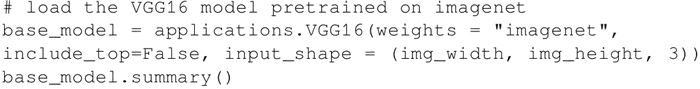上述代码的输出如下：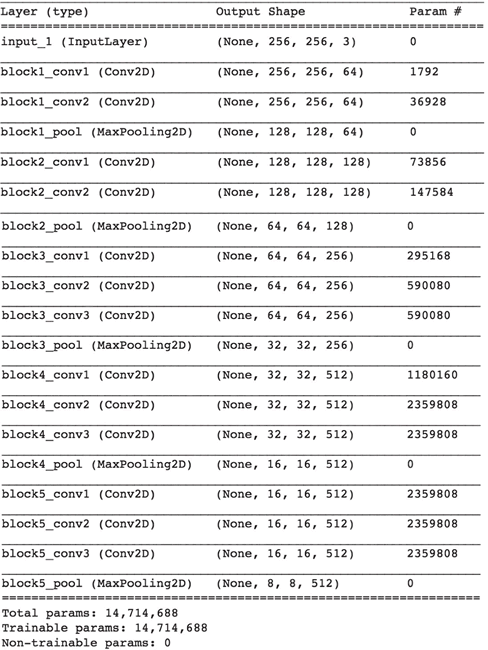1. 冻结预训练的 VGG16 网络的一定数量的较低层。在这里决定冻结最前面的 15 层：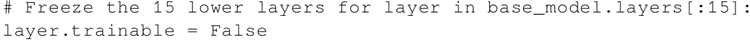2. 为了分类，添加一组自定义的顶层：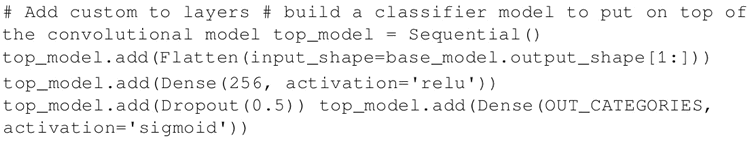3. 自定义网络应该单独进行预训练，为了简单起见，这里省略了这部分，将此任务交给读者：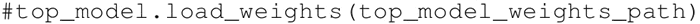4. 创建一个新的网络，这是预训练的 VGG16 网络和预训练的定制网络的组合体：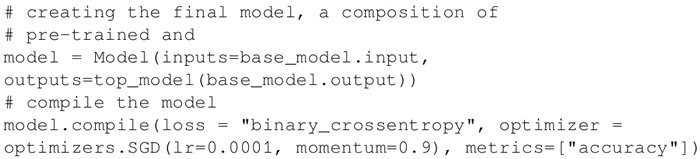1. 重新训练组合的新模型，仍然保持 VGG16 的 15 个最低层处于冻结状态。在这个特定的例子中，也使用 Image Augumentator 来增强训练集：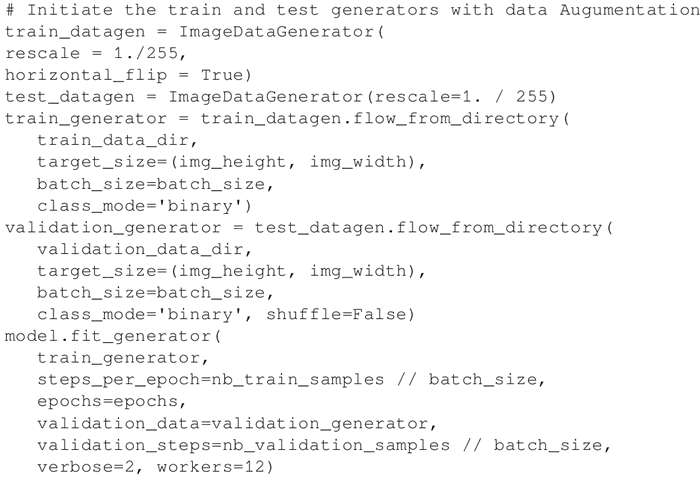1. 在组合网络上评估结果：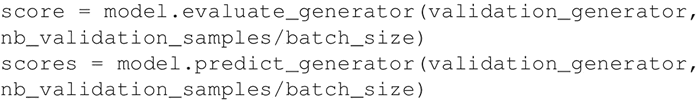解读分析
一个标准的 VGG16 网络已经在整个 ImageNet 上进行了预训练，并且使用了预先计算好的从网上下载的权值。这个网络和一个已经被单独训练的定制网络并置在一起。然后，并置的网络作为一个整体被重新训练，同时保持 VGG16 的 15 个低层的参数不变。

这个组合非常有效。它可以节省大量的计算能力，重新利用已经工作的 VGG16 网络进行迁移学习，该网络已经在 ImageNet 上完成了学习，可以将此学习应用到新的特定领域，通过微调去完成分类任务。

根据具体的分类任务，有几条经验法则需要考虑：

• 如果新的数据集很小，并且与ImageNet数据集相似，那么可以冻结所有的VGG16网络，并仅重新训练定制网络。这样，也可以最小化组合网络过度拟合的风险。
可运行代码 base_model.layers：layer.trainable=False 冻结所有低层参数。
• 如果新数据集很大，并且与ImageNet数据集相似，那么可以重新训练整个并置网络。仍然保持预先计算的权重作为训练起点，并通过几次迭代进行微调：
可运行代码 model.layers：layer.trainable=True 取消冻结所有低层的参数。
• 如果新数据集与ImageNet数据集有很大的不同，实际上仍然可以使用预训练模型的权值进行初始化。在这种情况下，将有足够的数据和信心通过整个网络进行微调。更多信息请访问http://cs231n.github.io/transfer-learning/。

展开全文• 由于 VGG16 并不是针对该问题而设计的，而是一个基于 ImageNET 上 1400 万张 1000 类图片而预训练的模型，在我的工作中只添加了必要的全连接层等，就实现了 95% 以上准确率的分类效果，可以说是较为满意的。...

# 1. 研究的问题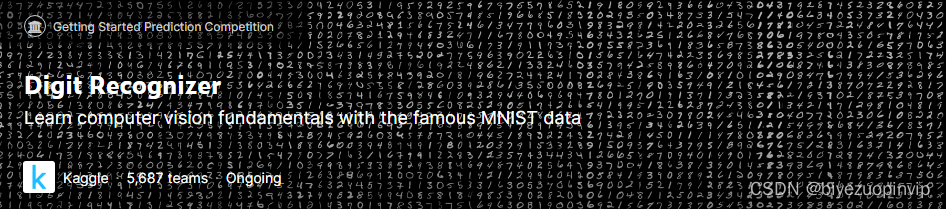该问题来源于 Kaggle 平台上的一个经典案例 Digit Recognizer ，目的是将数据集中的手写数字图片识别为数字。

在本次研究中，我简化了前人已有的模型，简要探究了迁移学习的基础方法，并将经典的预训练分类网络 VGG16 应用在数字识别中，对两者进行对比，以期达到相近的效果。

# 2. 问题的重要性和意义

该问题相当经典，也是入门神经网络的推荐选题，以及不少神经网络方面教程进行讲解的常用例子。但是经过了解，我认为推荐原因主要在于训练目标非常直观，而且数据集较小，使用非专业的设备也能进行模型训练，但是其背后的神经网络设计经常被一笔带过，但实际上仍较复杂。通常，该问题属于深度学习范畴，使用卷积神经网络(CNN)来解决。

传统方案的问题在于模型结构、参数需要完全手工设计。深度学习的核心是特征学习，旨在通过分层网络获取分层次的特征信息，因而需要理解常见结构在具体任务中的作用，如卷积、池化、全连接等，进行对比实验体会不同结构、参数对神经网络性能的影响，这个过程需要大量的先验经验，也是不同神经网络性能瓶颈的主要所在。除此之外，在训练时，所有参数都需要从完全未知的状态开始训练，训练时间长，往往需要多个 epochs 才能达到预期中较高的准确率。

基于此，我简要探究了迁移学习的方法。迁移学习是将已训练好的模型参数迁移到新的模型来帮助新模型训练，考虑到大部分数据或任务是存在相关性的，所以通过迁移学习可以将已经学到的模型参数，通过某种方式来分享给新模型从而加快并优化模型的学习效率，而不用像通常的传统网络设计流程那样从零学习。

因而我认为，研究该问题的意义在于，当需要应用神经网络处理某些问题时，例如将数字识别从传统的特征提取转变为分类问题，是否可以利用一种通用的预训练分类模型来简化神经网络设计的工作量，加快研究进度的同时能取得可接受的效果。

# 3. 前人工作

## 3.1 某个针对该问题设计的 CNN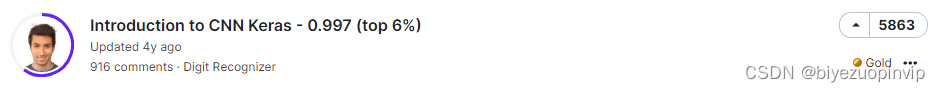我以 Kaggle 平台上最热门的开源解决方案为基础，其基于 Tensorflow 使用 Keras 搭建神经网络，模型结构如下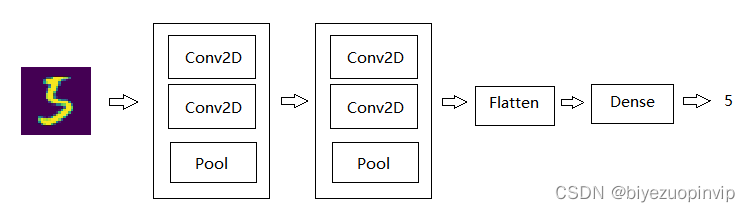# In -> [[Conv2D->relu]*2 -> MaxPool2D -> Dropout]*2 -> Flatten -> Dense -> Dropout -> Out
model = Sequential()

activation='relu', input_shape = (28, 28, 1)))
activation='relu'))

activation='relu'))
activation='relu'))
model.add(MaxPool2D(pool_size=(2, 2), strides=(2, 2)))



## 3.2 VGG16

常见的预训练分类网络有牛津的 VGG 模型、谷歌的 Inception 模型、微软的 ResNet 模型等，他们都是预训练的用于分类和检测的卷积神经网络(CNN)。

本次选用的是 VGG16 模型，是一个在 ImageNet 数据集上预训练的模型，分类性能优秀，对其他数据集适应能力优秀。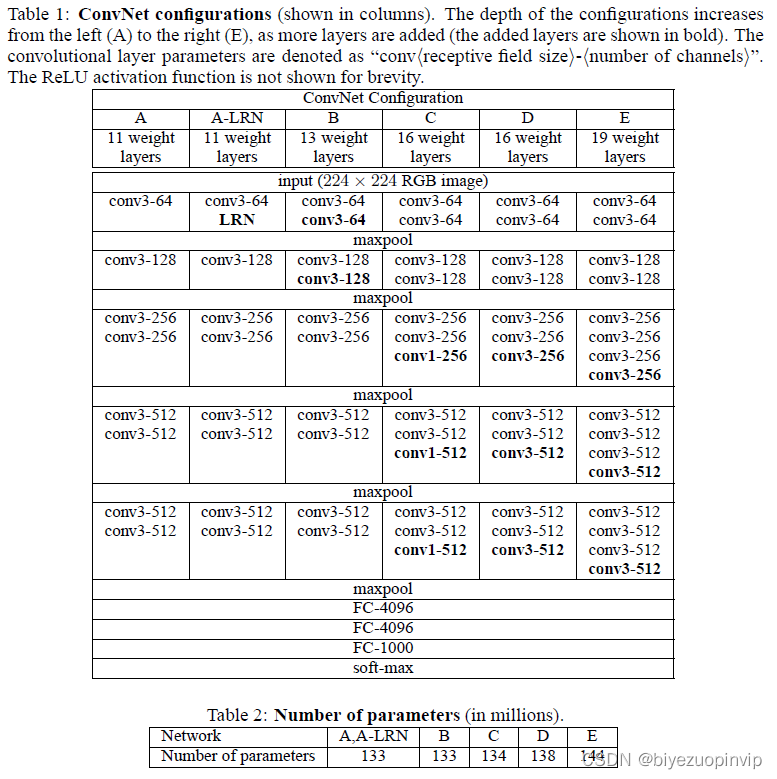上图为原论文中对 VGG16 模型内部结构的介绍，可以看出相当复杂，但在本次的研究中，并没有准备对该结构进行任何调整，而选择冻结其中所有的预训练参数，仅对这之后的几个必要层进行训练。

# 4. 解决方案

## 4.1 修改前人方案使用 Keras 的 MNIST 数据集

前人分享的代码使用的是 Kaggle 提供的 CSV 格式的数据集，将图片以像素为列，存储像素的灰度值。为了简化代码和方便验证两模型的准确率，统一使用 Keras 包中提供的数据集，训练集和测试集的获取方法如下

from keras.datasets import mnist
(X_train_data, Y_train_data), (X_test_data, Y_test_data) = mnist.load_data()


除此之外，原作者还设计了数据增强部分，在原数据集的基础上随机旋转、平移、缩放、产生噪音，从而更好地聚焦于数字特征的提取，而不是数据集本身。但受限于机器性能，为了缩减模型的训练时间，我删减了该部分功能。

通过如上修改，将该模型再与后续的基于 VGG16 的迁移学习模型进行比较，分析迁移学习得到的模型准确率水平。

## 4.2 VGG16+ 全连接层 迁移学习

我使用了 keras.applications.vgg16 中的 VGG16，在线获取已有的 VGG16 模型及参数，获取后冻结 VGG16 中的所有参数进行训练。

在这之后添加一层 relu 全连接以及用于多分类的 softmax 全连接，并插入卷积层到全连接层的过渡 flatten 层等，相较前人设计的 CNN 而言设计十分简要。

# In -> VGG16 -> Flatten -> Dense -> Dropout -> Dense -> Out
vgg16_model = VGG16(weights='imagenet', include_top=False, input_shape=(48, 48, 3))
for layer in vgg16_model.layers:
layer.trainable = False  # freeze VGG16卷积层的参数

model = Sequential()


## 4.3 分析比较模型结果

import numpy as np
import pandas as pd
import matplotlib.pyplot as plt
from keras.datasets import mnist

epochs = 1
test_total = 10000

df_CNN = pd.read_csv("./epochs%d/CNN.csv" % epochs)
df_VGG16 = pd.read_csv("./epochs%d/VGG16.csv" % epochs)

(X_train_data, Y_train_data), (X_test_data, Y_test_data) = mnist.load_data()
X_test_data = X_test_data.astype('float32') / 255.0
X_test = np.reshape(X_test_data, (-1, 28, 28, 1))
Y_test = Y_test_data

err_CNN = 0
err_VGG16 = 0

for i in range(test_total):
res_CNN = df_CNN["Label"][i]
res_VGG16 = df_VGG16["Label"][i]
if res_CNN != res_VGG16:
res_correct = Y_test[i]
if res_CNN != res_correct:
err_CNN = err_CNN + 1
if res_VGG16 != res_correct:
err_VGG16 = err_VGG16 + 1
plt.imshow(X_test[i][:, :, 0])
plt.savefig("./epochs%d/%d_%d_%d_%d.jpg" % (epochs, i, Y_test[i], res_CNN, res_VGG16))

print(err_CNN, err_VGG16)


训练 epochs=1 后，通过以上代码，输出两模型对于分类结果预测不一致的测试样例，命名为”样例序号_参考结果_前人 CNN 预测结果_VGG16 预测结果.jpg”，并且输出 10000 个测试样例中的错误分类数，输出及部分样例如下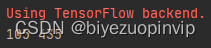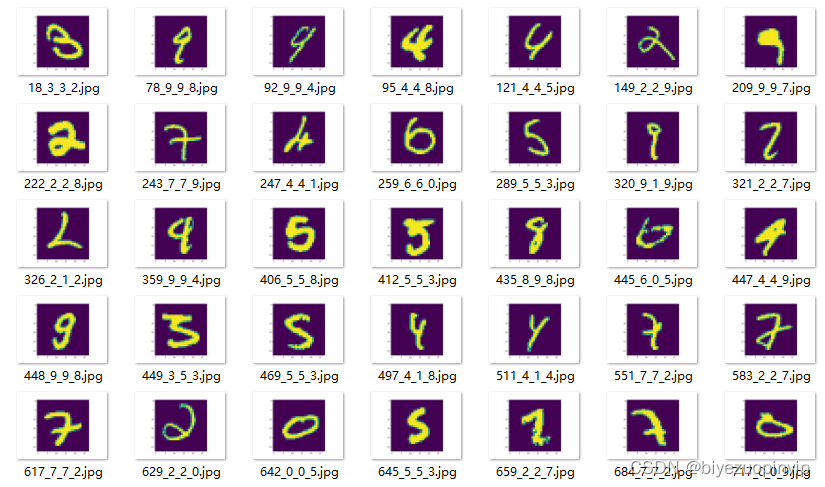观察如上结果，在 10000 张测试样例中，前人 CNN 准确率为 98.95%，应用了 VGG16 进行迁移学习的模型准确率为 95.65%，虽然结果不及 CNN，但是我认为这已经超过了我预期的结果。

## 4.4 总结

由于 VGG16 并不是针对该问题而设计的，而是一个基于 ImageNET 上 1400 万张 1000 类图片而预训练的模型，在我的工作中只添加了必要的全连接层等，就实现了 95% 以上准确率的分类效果，可以说是较为满意的。

通过观察如上错误样例，能够发现 VGG16 将一些形状十分类似于另一数字的图片分类成了另一数字，例如右半部分较短、下半部分较长的”4”分类成了”9”，将下半部分极窄的”8”分类成了”9”，能够感受到 VGG16 更多的是在将形状类似的图片分为一类，而并没有像 CNN 那样通过(5,5)、(3,3)的 kernel 聚焦于数字的特征，这在对于分类一些书写并不规范且特殊的数字而言是致命的，但对于正常数字识别而言是能够接受的，要解决该问题，可能需要调整 VGG16 中的内部结构。

# 5. 用于验证方法的数据集

## 5.1 MNIST

将数据集中的像素信息转化为图片，由于实际训练中不需要图片信息，在此我仅将测试集的一部分样例转化为图片进行演示，代码如下

for i in range(100):
plt.imshow(X_test[i][:, :, 0])
plt.savefig("./test/%d.jpg" % i)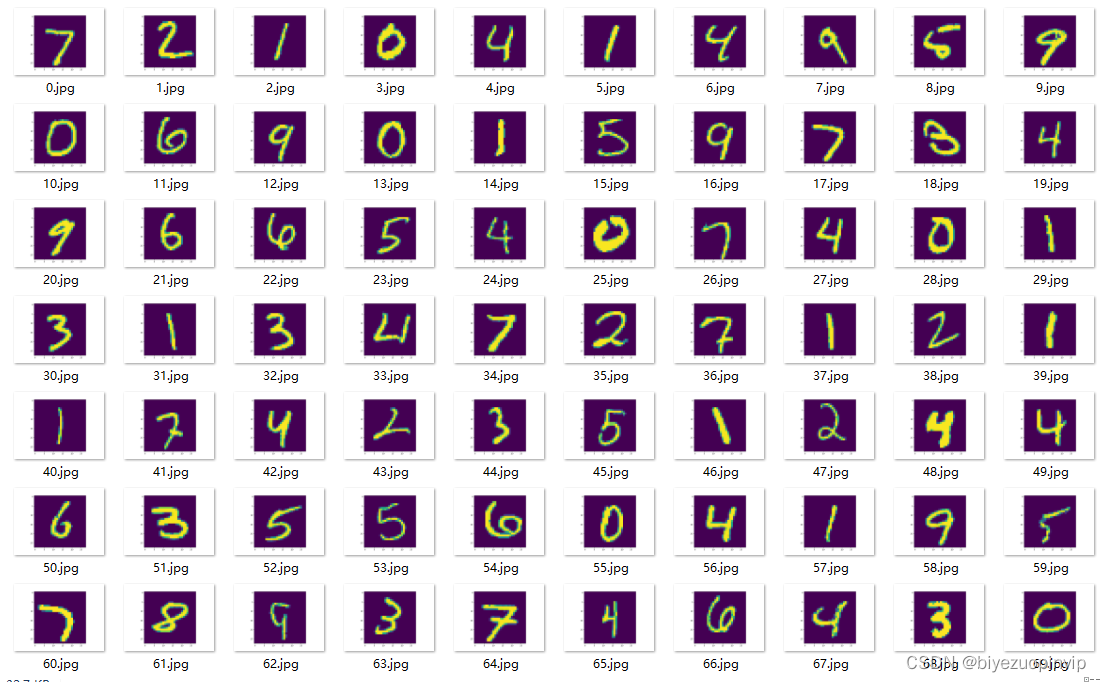展开全文python 迁移学习 基于VGG16
• VGG16迁移学习问题 我把特征提取层冻结掉了，更改了全连接层做了训练，为了做对比，那现在我想只冻结前四个卷积块，把最后一个解冻和全连接层一起更改，应该要怎么写啊，求大虾们帮帮忙QAQ import numpy as np ...pytorch 迁移学习 神经网络
• keras+vgg16迁移学习实践自己划分的数据集； 关联博客：https://blog.csdn.net/qq_31681523/article/details/115758878迁移学习
• VGG16迁移学习 keras框架 backend:theano 更改文件中的图片路径 和输出权重路径 需要另外下载imagenet1000权重
• 用Keras和VGG16模型转移学习。 使用的数据集是从网站获取：https://www.kaggle.com/moltean/fruits 不要忘记修改数据集的路径。
• VGG16迁移学习 from __future__ import print_function,division import torch import time import torch.nn as nn import torch.nn.functional as F import torch.optim as optim from torchvision import datasets...pytorch 图像处理
• VGG16网络进行迁移学习用来进行图像的多分类
• 人工智能-项目实践-迁移学习-使用VGG19迁移学习实现图像风格迁移 使用VGG19迁移学习实现图像风格迁移 这是一个使用预训练的VGG19网络完成图片风格迁移的项目，使用的语言为python，框架为tensorflow。 给定一张...
• import tensorflow as tf ...from tensorflow.keras.applications.vgg16 import VGG16 class myNet: def init(self): #对于训练用数据集，使用了数据增强 self.generatorForTrain = tf.keras.preprocessing.image.Ima迁移学习 keras
• 由于深度学习网络的训练复杂，耗时，并且对硬件要求较高，因此对于目标相似的任务，并且自己的数据集数目较小的时候，比如分类任务，不同的分类任务之间的对象具有较为相似的特征，我们...这里我们采用VGG16来实现简单tensorflow python
• 什么是迁移学习？我们总是被告知“熟能生巧”，我们被要求在不同领域练习大量问题，为期末考试做好准备。我们解决的问题越多，我们就越能更好地利用这些知识以解决新问题。如果有一种方法可以应用相同的...神经网络 python 机器学习 人工智能
• Pytorch迁移学习训练VGG16和模型测试代码（采用华为云modelarts训练），训练猫狗分类模型
• 利用VGG16网络模块进行迁移学习，实操（附源码）-附件资源
• 在这个小项目中，我们将迁移VGG16网络来训练Kaggle的猫与狗数据集。目标是能让我们迁移学习出来的网络可以高效的识别训练和测试集中的猫与狗。数据集可以直接移步到kaggle的官方网站上下载。传送门 2. 迁移学习 迁移...机器学习 python 人工智能 计算机视觉
• 主要介绍了keras实现VGG16 CIFAR10数据集方式，具有很好的参考价值，希望对大家有所帮助。一起跟随小编过来看看吧
• 迁移学习 Transfer Learning ——猫狗分类（PyTorch）3.2 迁移 VGG163.2.1 通过代码自动下载模型并直接调用3.2.2 当前迁移过来的模型进行全连接层的调整3.2.3 模型训练及结果 前文关于迁移学习的入门及自定义模型的...pytorch 迁移学习 神经网络 计算机视觉
• 个人简介：李响Superb，CSDN百万访问量博主，普普通通男大学生，目前在读软件工程，计算机视觉、深度学习和医学图像处理专攻，偶尔也搞迁移学习和全栈开发。 博客地址：lixiang.blog.csdn.net 文章目录1 前言2 ...迁移学习 分类
•迁移学习 分类 pytorch
• 1.输出效果： 实际的图片——Dog： 预测的结果有误。但是没关系，自己可以找一些数据...import tensorflow.keras.applications.vgg16 from PIL import Image from tensorflow import keras from tensorflow.keras.prpython 计算机视觉
• 使用VGG19迁移学习实现图像风格迁移 这是一个使用预训练的VGG19网络完成图片风格迁移的项目，使用的语言为python，框架为tensorflow。 给定一张风格图片A和内容图片B，能够生成具备A图片风格和B图片内容的图片C。此...
• 本程序基于VGG16的进行迁移学习，通过训练自己的数据集实现三分类，通过冻结原网络的特征提取层，使卷积层和池化层的权重保持不变。由于待分类的数据与原先 VGG16 的分类数据不同，删除原来的全连接层，在特征提取层...迁移学习 神经网络 python tensorflow
• 这是一个使用预训练的VGG19网络完成图片风格迁移的项目，使用的语言为python，框架为tensorflow。 给定一张风格图片A和内容图片B，能够生成具备A图片风格和B图片内容的图片C。
• 利用VGG16对kaggle比赛提供的猫狗图片进行迁移学习-PyTorch版本 1.已改写为gpu-cpu通用 2.数据集处理参考注释；含后续训练、训练集/验证集accuracy计算，图片测试 3.数据集请参考...
• Vgg16的基本网络结构 Vgg16的网络结构由13层卷积层+3层全连接层组成 kaggle上猴子的数据源：https://pan.baidu.com/s/1Y15Tsm_hmP6pMHnGpOA4qg提取码：6ass 首先通过torchvision导入模型vgg16(也可以通过...pytorch...